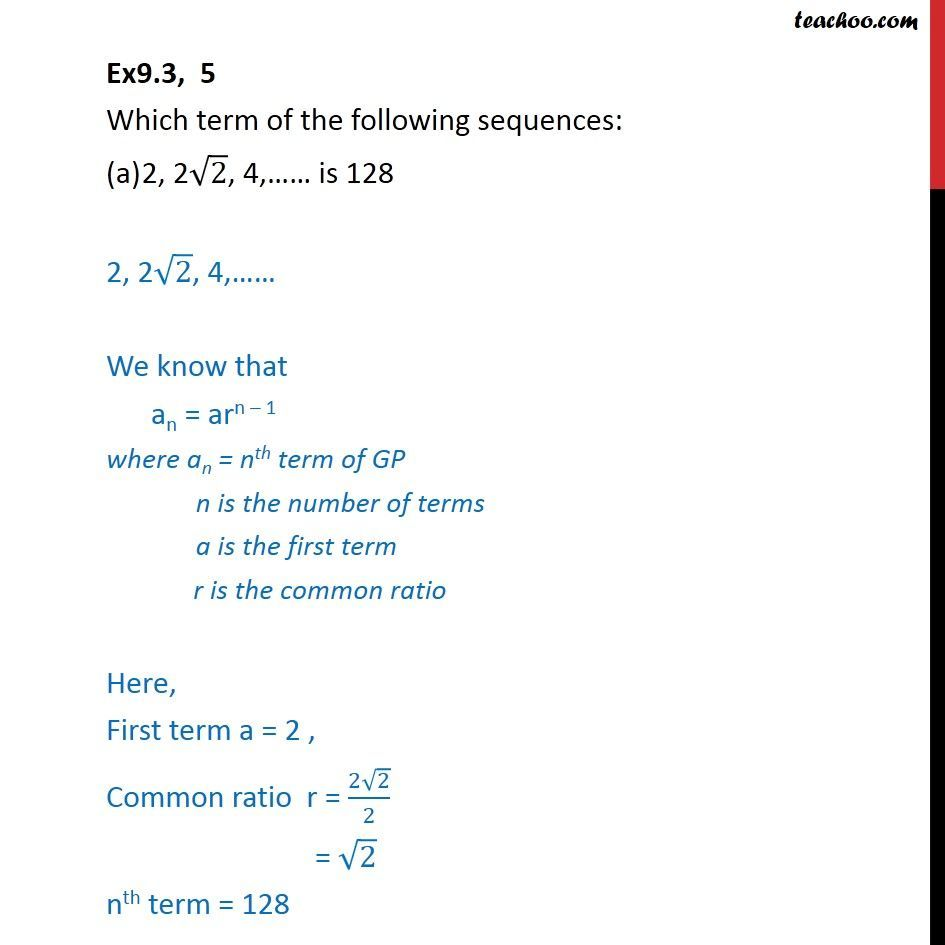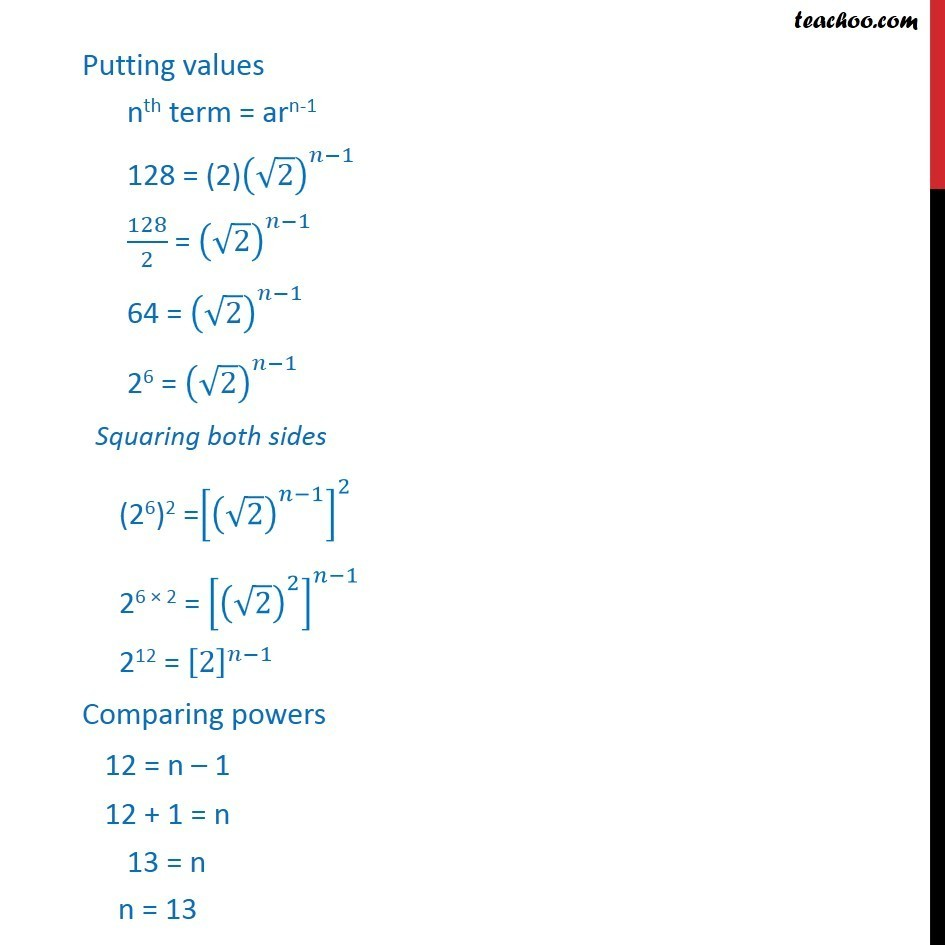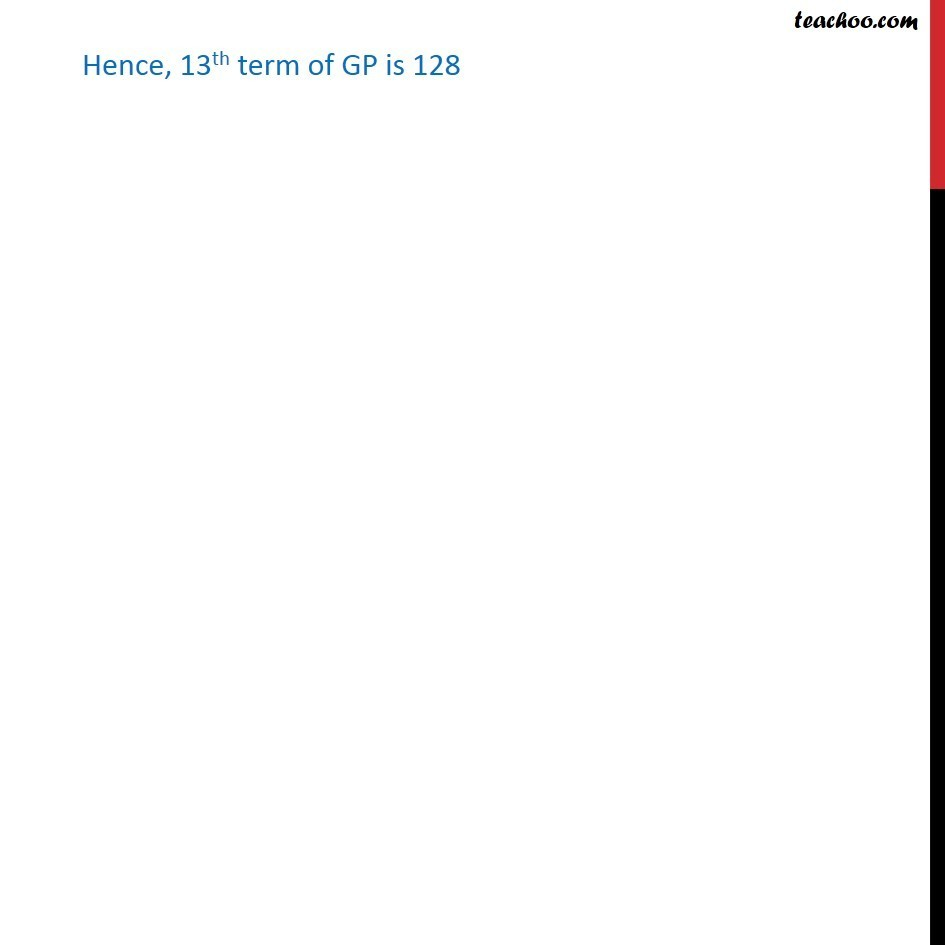Geometric Progression(GP): Formulae based

Chapter 8 Class 11 Sequences and Series
Concept wiseLearn in your speed, with individual attention - Teachoo Maths 1-on-1 Class

### Transcript

Ex9.3, 5 Which term of the following sequences: 2, 2 2, 4, is 128 2, 2 2, 4, We know that an = arn 1 where an = nth term of GP n is the number of terms a is the first term r is the common ratio Here, First term a = 2 , Common ratio r = (2 2)/2 = 2 nth term = 128 Putting values nth term = arn-1 128 = (2)( 2)^( 1) 128/2 = ( 2)^( 1) 64 = ( 2)^( 1) 26 = ( 2)^( 1) Squaring both sides (26)2 =[( 2)^( 1) ]^2 26 2 = [( 2)^2 ]^( 1) 212 = ^( 1) Comparing powers 12 = n 1 12 + 1 = n 13 = n n = 13 Hence, 13th term of GP is 128